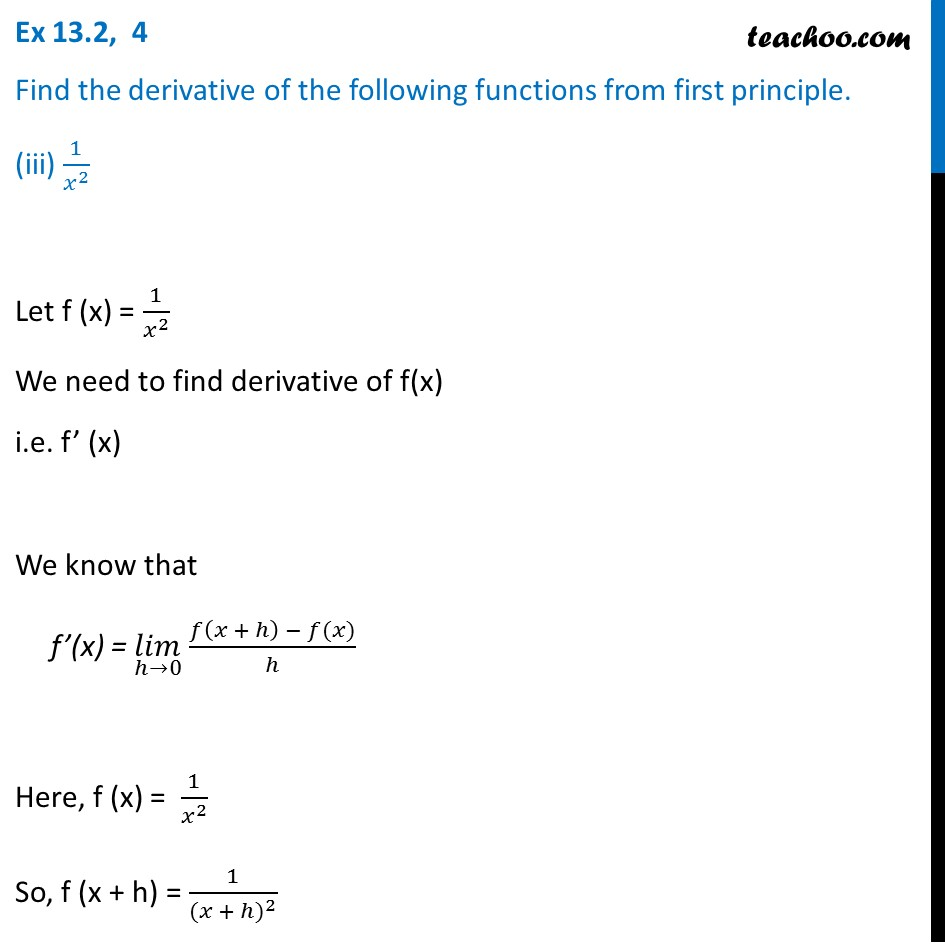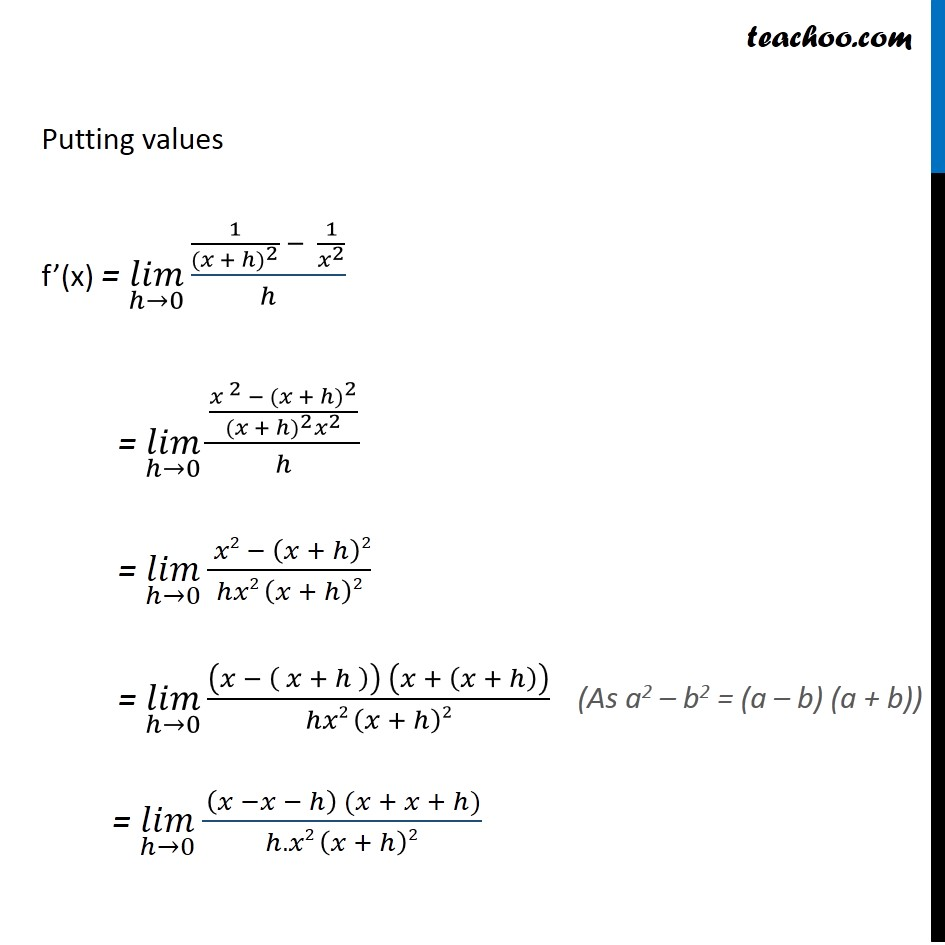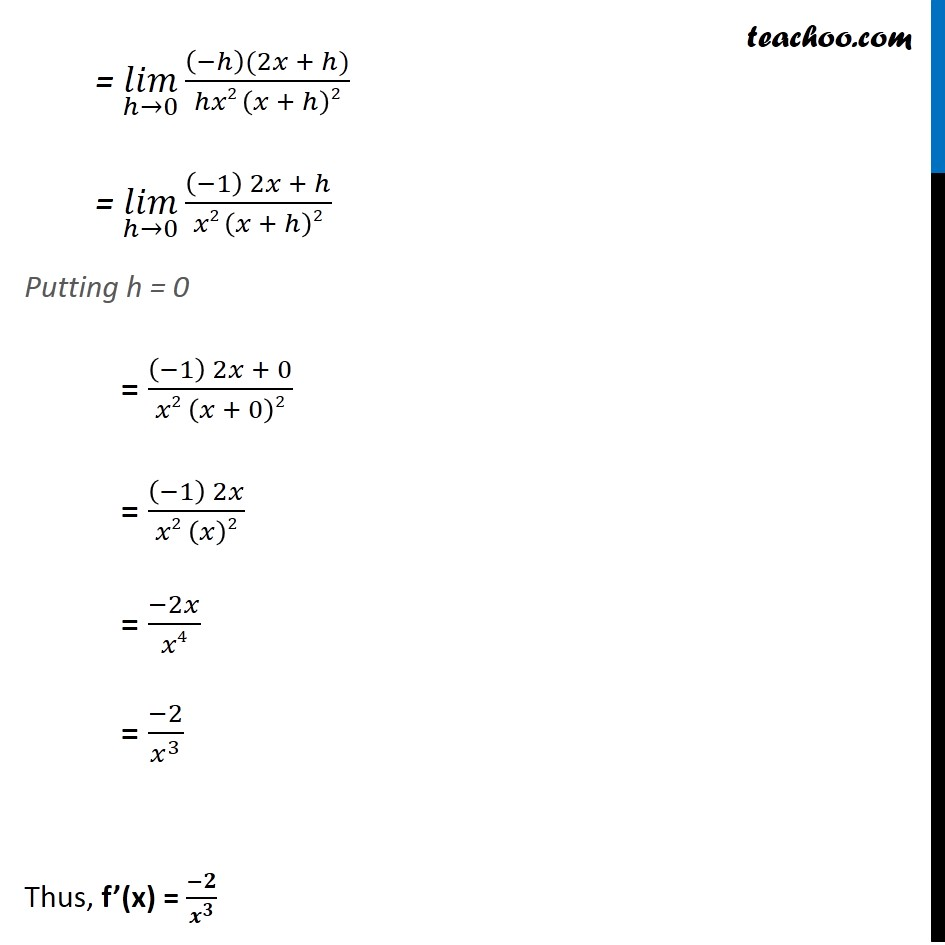Ex 12.2

Chapter 12 Class 11 Limits and Derivatives
Serial order wiseLearn in your speed, with individual attention - Teachoo Maths 1-on-1 Class

### Transcript

Ex 12.2, 4 Find the derivative of the following functions from first principle. (iii) 1/𝑥^2 Let f (x) = 1/𝑥^2 We need to find derivative of f(x) i.e. f’ (x) We know that f’(x) = (𝑙𝑖𝑚)┬(ℎ→0) 𝑓⁡〖(𝑥 + ℎ) − 𝑓(𝑥)〗/ℎ Here, f (x) = 1/𝑥^2 So, f (x + h) = 1/〖(𝑥 + ℎ)〗^2 Putting values f’(x) = (𝑙𝑖𝑚)┬(ℎ→0)⁡〖(1/〖(𝑥 + ℎ)〗^2 − 1/𝑥^2 )/ℎ〗 = (𝑙𝑖𝑚)┬(ℎ→0)⁡〖(〖〖𝑥 〗^2 − (𝑥 + ℎ)〗^2/(〖(𝑥 + ℎ)〗^2 𝑥^2 ))/ℎ〗 = (𝑙𝑖𝑚)┬(ℎ→0)⁡〖( 𝑥2 − (𝑥 + ℎ)2)/(ℎ𝑥2 (𝑥 + ℎ)2)〗 = (𝑙𝑖𝑚)┬(ℎ→0)⁡〖((𝑥 − ( 𝑥 + ℎ )) (𝑥 + (𝑥 + ℎ)))/(ℎ𝑥2 (𝑥 + ℎ)2)〗 = (𝑙𝑖𝑚)┬(ℎ→0)⁡〖( (𝑥 −𝑥 − ℎ) (𝑥 + 𝑥 + ℎ))/(ℎ.𝑥2 (𝑥 + ℎ)2)〗 (As a2 – b2 = (a – b) (a + b)) = (𝑙𝑖𝑚)┬(ℎ→0)⁡〖((−ℎ)(2𝑥 + ℎ))/(ℎ𝑥2 (𝑥 + ℎ)2)〗 = (𝑙𝑖𝑚)┬(ℎ→0)⁡〖((−1) 2𝑥 + ℎ)/(𝑥2 (𝑥 + ℎ)2)〗 Putting h = 0 = ((−1) 2𝑥 + 0)/(𝑥2 (𝑥 + 0)2) = ((−1) 2𝑥)/(𝑥2 (𝑥)2) = (−2𝑥)/𝑥4 = (−2)/𝑥^3 Thus, f’(x) = (−𝟐)/𝒙^𝟑